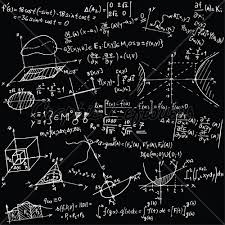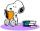# Simple equation 5

Solve equation with fractions: X × 3/8 = 1/2

X =  1.3333

### Step-by-step explanation:

X • 3/8 = 1/2

3X = 4

X = 4/3 ≈ 1.333333

Our simple equation calculator calculates it.Did you find an error or inaccuracy? Feel free to write us. Thank you!Tips to related online calculators
Need help to calculate sum, simplify or multiply fractions? Try our fraction calculator.
Do you have a linear equation or system of equations and looking for its solution? Or do you have a quadratic equation?

## Related math problems and questions:

• Equation 25Solve following simple equation: 3/4(x+5)=1/2(x+9)
• Simple equation 324 = n • 27, solve for n
• Simple equationSolve the following simple equation: 2. (4x + 3) = 2-5. (1-x)
• Simple equation 2Find X in this simple equation: X/9 = 96/108
• Simple equation 9Solve the following equation: -8y+5=-9y+9
• Simple equation 8Solve the following equation: 36=-(1+7x)-6(-7-x)
• Equation 15Solve equation with variables on both sides:
• Simple equation 6Solve equation with one variable: X/2+X/3+X/4=X+4
• Equation 11Solve equation: 0=y-1,2.(y-1,5)
• EquationSolve equation and check the result: 1.4x - 3/2 + x - 9,8 = x + 0,4/3 - 7 + 1,6/6
• Find xSolve: if 2(x-1)=14, then x= (solve an equation with one unknown)
• Reciprocal equation 3Solve reciprocal equation: 1/2 + 2/3=1/x
• EquatiomSolve equation with negatives: X/(-5) + 2 = -9
• Equation algebraogramSolve the equation: oco + ivo = cita How much has the task of solutions?
• Fraction + eqSolve following simple equation with fractions: -5/6(8+5b) = 75 + 5/3b
• FruitsAmy bought a basket of fruits 1/5 of them were apples,1/4 were oranges, and the rest were 33 bananas. How many fruits did she buy in all?
• Equation with fractionsSolve an equation: 1- (2x-5)/(6) =3- (x)/(4) It is an equation with fractions.